Courses

# Test: Solid State

## 25 Questions MCQ Test Chemistry for JEE | Test: Solid State

Description
This mock test of Test: Solid State for JEE helps you for every JEE entrance exam. This contains 25 Multiple Choice Questions for JEE Test: Solid State (mcq) to study with solutions a complete question bank. The solved questions answers in this Test: Solid State quiz give you a good mix of easy questions and tough questions. JEE students definitely take this Test: Solid State exercise for a better result in the exam. You can find other Test: Solid State extra questions, long questions & short questions for JEE on EduRev as well by searching above.
QUESTION: 1

### Substances that are strongly attracted by applied magnetic field and can be permanently magnetized are

Solution:

Ferromagnetic substance get permanently magnetised in applied Magnetic Field.

QUESTION: 2

### Which type of solid conduct electricity in molten state but not in solid state?

Solution:

In molten state ions can exist so they can conduct electricity

QUESTION: 3

### Yellow colour of NaCl crystals in sodium vapour is due to

Solution:
• This yellow colour is due to metal excess defect of anionic valency.
• Na+ion deposited on the surface of crystal of NaCl . The Cl-ion diffused to form NaCl. This happens by loss of electron from Na to Na+.
• The released electro diffused into the crystal and occupy anionic site called F center which imparts yellow colour to NaCl.
QUESTION: 4

Dislocation defect is also known as

Solution:

In frenkel defect cation gets dislocated from its position.

QUESTION: 5

Defects in solids can be studied using

Solution:

All of the above mentioned methods are used to detect the basic structure of the sample, hence can easily detect the defects present in a sample.

QUESTION: 6

Point defect is also known as

Solution:

Point defects are zero dimensional defects as they cannot extend in any direction in space. Point defects occur where an atom is missing or misplaced in a crystal lattice. The limit of the size of point defect is not defined.

QUESTION: 7

Solid X is a very hard electrical insulator in solid as well as in molten state. It melts at extremely high temperature. Solid X is a

Solution:

Covalent solid doesnot conduct electricity in molten and solid state.

QUESTION: 8

Nature of binding forces present in carbondioxide molecules in solid state are?

Solution:

O=C=O<−>−O−C+=O

QUESTION: 9

What type of interaction hold the molecules together in a polar molecular solid?

Solution:

Polar molecule will act as a dipole.

QUESTION: 10

An atom located at the body center of a cubic unit cell is shared by

Solution:

In body centred cubic cell, an atom at the body-centre is shared by 1 unit cell. Thus, this atom contributes one atom to the unit cell.

QUESTION: 11

Ionization enthalpy is lowest in

Solution:

Metals have low ionization energy because of there tendency to lose electrons.

QUESTION: 12

The coordination number of AB having rock salt geometry is

Solution:

NaCl has rock salt structure. Coordination No. is 6.

QUESTION: 13

MgO has the structure of NaCl. The coordination number of the ions in MgO is

Solution:

Coordination No. in NaCl is 6.

QUESTION: 14

Type of stoichiometric defect shown by ZnS is

Solution:

ZnS shows Frenkel defect.

QUESTION: 15

A metal crystallizes with a face-centered cubic lattice. The edge of the unit cell is 408 pm. The diameter of the metal atom is

Solution: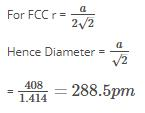QUESTION: 16

An element forms a cubic unit cell with edge length 405 pm. Molar mass of this element is 2.7 X 10-2 Kg/mol and its density is given as 2.7 X 10Kg/m3. How many atoms of this elements are present per unit cell.

Solution: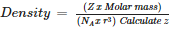QUESTION: 17

A group of 14 element is converted into n – type semiconductor by dopping it with

Solution:

Group 15 doping on group 14 element forms n type semiconductor due to excess electrons.

QUESTION: 18

Group 14 element is converted to p – type semiconductor by dopping it with

Solution:

Doping of group 14 elements with group 13 forms p type semiconductor.

QUESTION: 19

Giant ionic structures is also name given to

Solution:
• An ionic compound is a giant structure of ions.
• The ions have a regular, repeating arrangement called an ionic lattice .
• The lattice is formed because the ions attract each other and form a regular pattern with oppositely charged ions next to each other.
QUESTION: 20

A substance forms face centered cubic crystals. Its density is 1.984 g/cm3 and the length of the edge of the unit cell is 630 pm. Calculate the molar mass in g/mol?

Solution: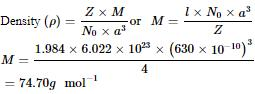QUESTION: 21

The hardest substance among the following is

Solution:

It has network Structure.

QUESTION: 22

The following diagram shows :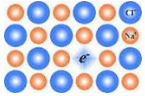Solution:

Extra electron is present at anionic site.

QUESTION: 23

In n – type semiconductor current is carried by

Solution:

N type semiconductors have excess electrons.

QUESTION: 24

The following diagram shows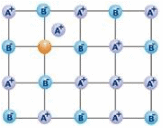Solution:

A Frenkel defect or dislocation defect is a type of point defect in crystalline solids named after its discoverer Yakov Frenkel. The defect forms when an atom or smaller ion (usually cation) leaves its place in the lattice, creating a vacancy, and becomes an interstitial by lodging in a nearby location.

QUESTION: 25

Which transition metal oxide has appearance and conductivity like that of copper?

Solution:

Rhenium oxide and cooper oxide have same appearance and conductivity.